# Introduction to Spectral Analysis

In this assignment, we will look at the basics of spectral analysis. Appropriately for oceanography, we will work with complex-valued velocity data, and will show how the spectrum can be thought of either as rotary components (positive and negative), or as Cartesian components (eastward and westward). In general, gaining a good understanding of a system requires using both, as different features are more clear from one perspective or other.
As before, we will work with the Bravo mooring from the central Labrador Sea. We'll look at the deepest depth. We will go through this kind of slowly to appreciate some of the subtleties involved. As before, this is intended to give you a practical introduction to creating these spectra; the theoretical understanding will come later.

# Topics Covered

The topics covered in this assignment are
1. Computing the periodogram from the magnitude-squared Fourier transform
2. Computing the periodogram using mspec
3. Changing axis scalings using ylin, ylog, xlin, and xlog
5. Finding the Coriolis and tidal frequencies with corfreq and tidefreq
6. Multitaper spectral estimation using mspec and sleptap
7. Balancing bias and variance
8. Rotary spectra
9. Cartesian spectra and rotated Cartesian spectra

# The Periodogram, a.k.a. the Naive Spectral Estimate

The first point to know is that the spectrum is not something that can be computed. Instead, it is estimated. The true spectrum is a theortical object that can't be computed unless you have an infinite amount of time and perfect sampling. The things that can be computed are called spectral estimates or estimated spectra. Later, we will discuss in detail more the difference between the true spectrum and spectral estimates.
Firstly, we will look at the modulus-squared Fourier transform. It is common for this to be referred to as ‘the spectrum’. However, this terminology is incorrect and misleading. Instead, the modulus-squared Fourier transform is a type of spectral estimate known as the periodogram. It is known, actually, to be a very poor spectral estimate for reasons we will learn about later. Note that it is generally a good idea to remove the mean when computing spectral estimates.
use bravo94.rcm
cv=cv(:,6); cv=cv-mean(cv);
clf,
plot(abs(fft(cv)).^2),axis tight,ylog,ylim(10.^[2 8])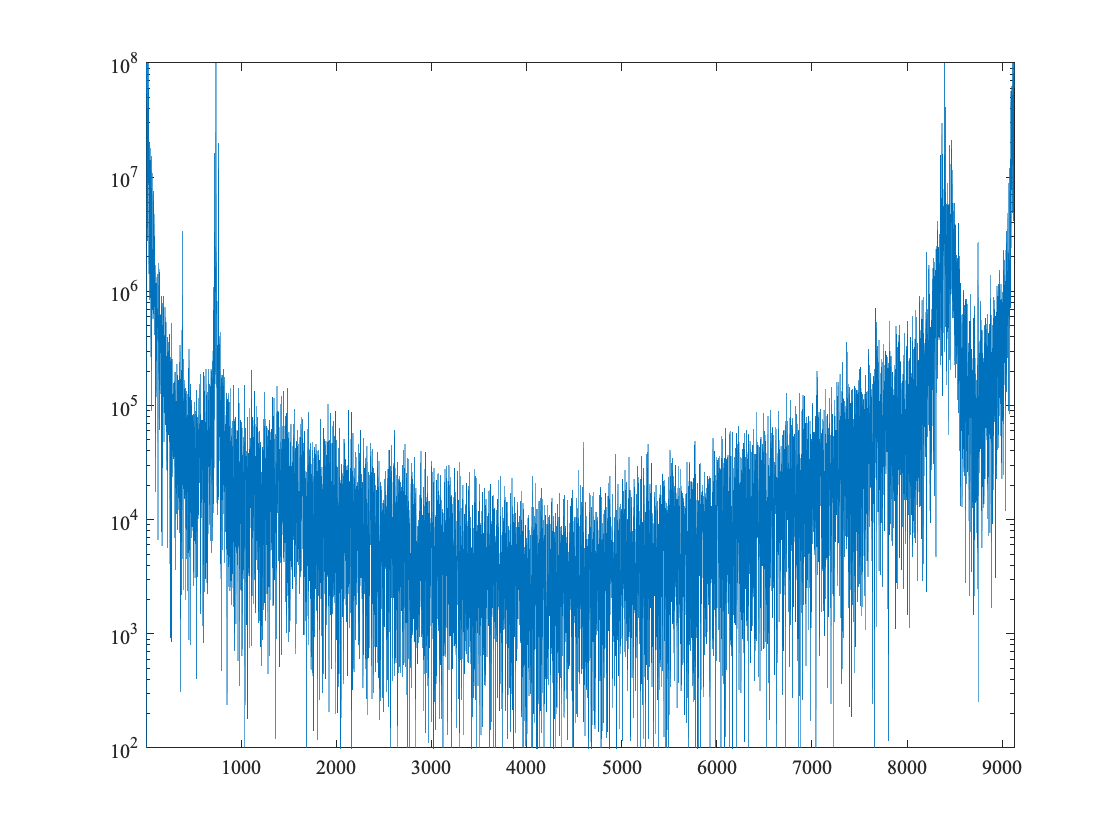Here ylog is a jLab function to set the y-axis to be logarithmic. Related functions are ylin, xlin, and xlog. Take a moment to evaluate the above plot command in the Matlab command window and play around with changing the axes from linear to logarithmic in this way. In doing so, you might want to dock the figure window by clicking on the curved arrow on the right-hand side of the menu bar.
In the array output by fft, positive frequencies (circles rotating in a counterclockwise sense) are on the left, while negative frequencies (circles rotating in a clockwise sense) are on the right, appearing in reverse order as we have discussed in class. Thus the highest resolvable frequency, called the Nyquist frequency, is in the middle.
You can see clearly that the periodogram has a certain symmetry if you reflect it about the middle. However, it is not completely symmetric because, with a complex-valued signal such as velocity, the twin frequencies (positive and negative rotations at the same absolute frequency) are not required to cancel.
The bump on the right is the internal wave peak, localized around the inertial frequency. These primarily rotate in a clockwise or mathematically negative sense; they do not exhibit any significant contribution from counterclockwise rotating frequencies. The line we see on the left is from the tides; it also occurs on the right, but is obscured by the inertial peak. We will see all of this more clearly soon.
Compare this with the periodogram of just the eastward velocity:
clf,
plot(abs(fft(real(cv))).^2),axis tight,ylog,ylim(10.^[2 8])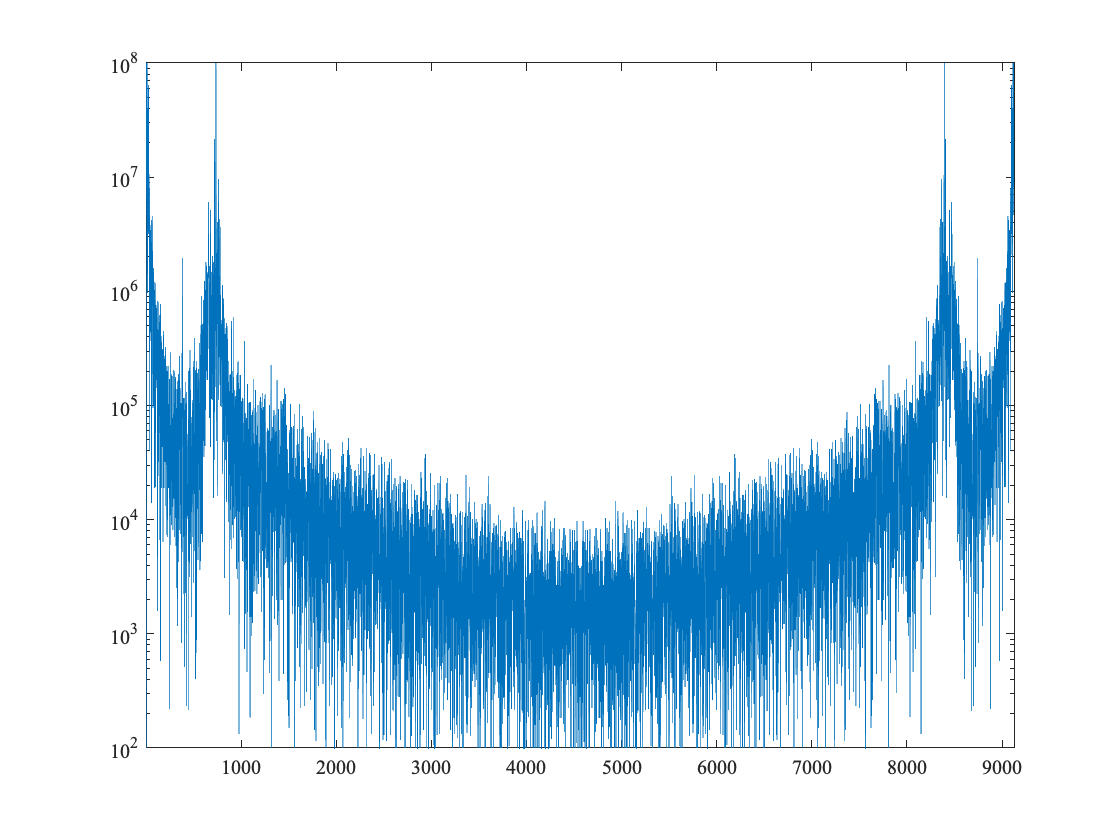Now, the periodogram is perfectly symmetric about the center or Nyquist frequency. As we discussed in class, twin frequencies occur in conjugate pairs, with the same magnitudes but reversed phase. Because of this, two complex exponentials rotating in opposite directions cancel to yield a real-valued, phase-shifted sinuosoid. The symmetry we see in the periodogram is a reflection of the fact that the time series is real-valued.
It will be seen later that adding two oppositely-rotating circles in general leads to an ellipse. When the magnitude of one circle vanishes the result is obviously a circle. When the magnitude of both circles are identical, the result is a line, and this is the case for a real-valued time series.

# One-Sided Spectra

There are several inconvenient aspects of computing the periodogram in this way. Firstly, it is a little bit of a hassle to figure out where the frequencies are. Secondly, it is a bit odd to see the positive and negative frequencies meet in the middle at the Nuquist. Thirdly, the y-axis should be normalized in a meaningful way.
These issues are all addressed using the jLab function mspec, which computes so-called one-sided spectral estimates. The main function of mspec is to compute a type of spectral estimate called the mutli-taper spectral estimate, hence the ‘m’. However it can also compute the periodogram, which we will do first.
clf,
[f,spp,snn]=mspec(1,cv,[]);
plot(f,[spp snn]),axis tight,ylog,ylim(10.^[-2 5])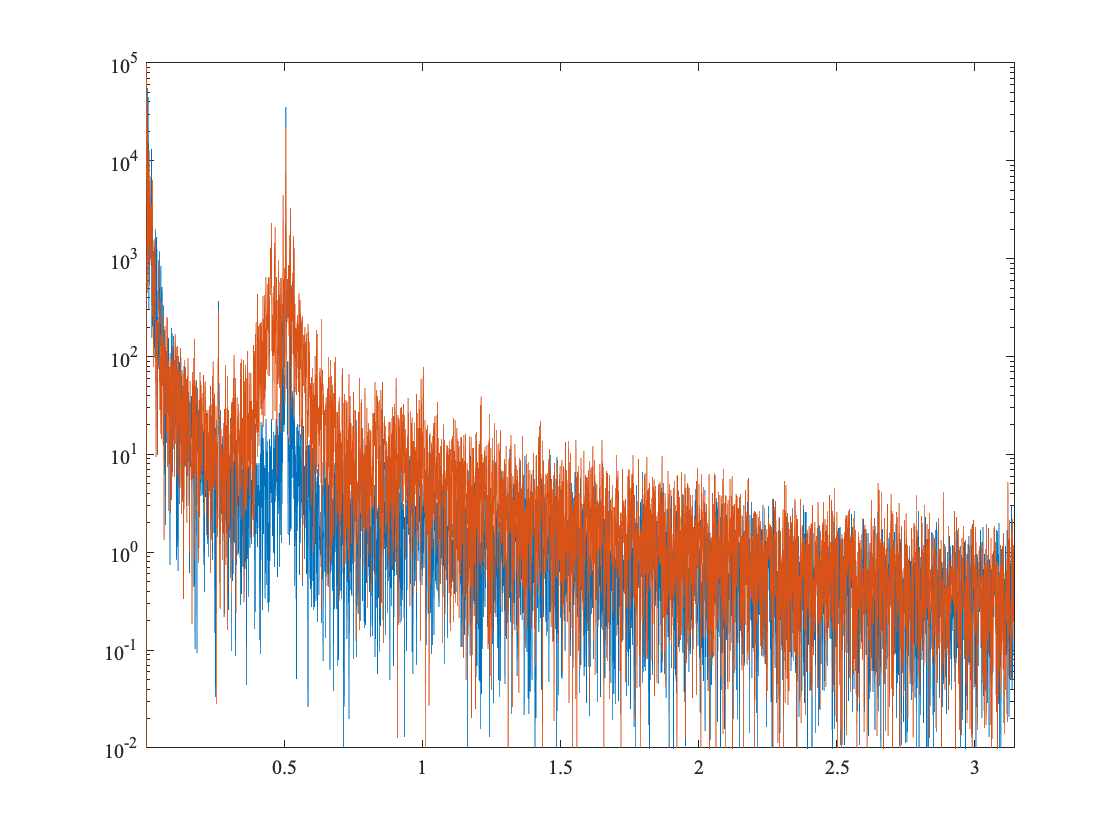The ‘1’ as the first argument is the sampling interval, in hours, while the empty array ‘[]’ as the third argument specifies that the periodogram is to be computed.
Here the two-sided periodgram has been split into two one-sided portions, spp and snn, each containing roughly half as many data points as the original time series. Blue is for positive (counterclockise) rotations, while orange is for negative (clockwise) rotations. These are hard to see at the moment; we will get to that later.
mspec has also normalized the y-axis in a sensible way. As we will see later, the spectrum integrates to the variance. For the two-sided periodgram with an odd number of points, the correct formula is
(1/2/pi)*(f(2)-f(1))*sum(spp(2:end)+snn(2:end))
ans =
9.732892801337762e+01
std(cv,1).^2
ans =
9.732890655162268e+01
in which we sum over all frequencies, omitting the first, the zero frequency, from both portions. The f(2)-f(1)is a differential, as would appear in an intergal. It is seen that the summing over the spectrum in this way, we obtain the variance as computed in the time domain.

Next we put down some markers of some meaningful frequencies
clf,
[f,spp,snn]=mspec(1,cv,[]);
plot(f,[spp snn]),axis tight,ylog,ylim(10.^[-2 5])
vlines(corfreq(lat),'2G--'),vlines(tidefreq,':')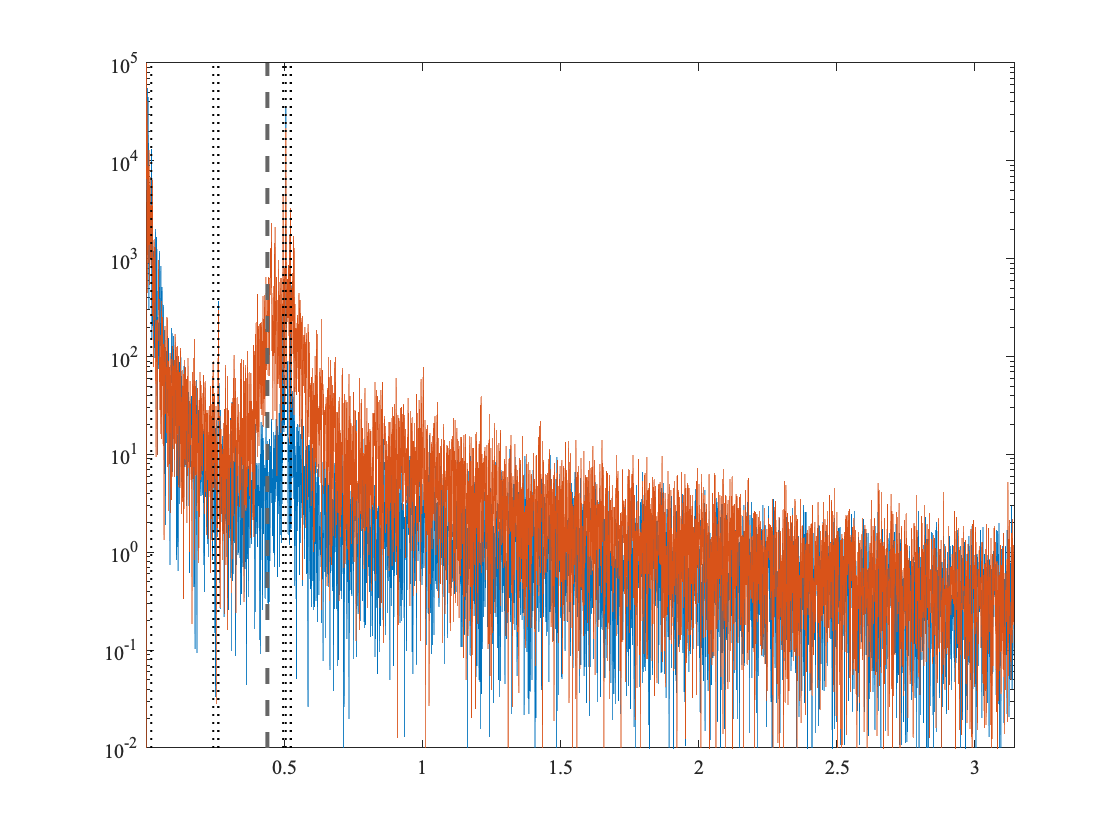Here we are using vlines to plot vertical lines corresponding to the local Coriolis frequency (heavy dashed line), and the eight major tidal components (dotted lines). These are computed using the jLab routines corfreq and tidefreq respectively, which return these frequencies in units of radians per hour. The tidal frequencies appear in three groups. From right to left, there are four semidiurnal tidal frequencies, three diurnal frequencies, and one low-frequency tide, the Mf lunal fortnightly tide at about one cycle per 13.6 days. tidefreq can also outputs individual tidal components; you might want to quickly take a look at the help for this function.
As we have discussed, when a frequency is expressed in units of radians per unit time, it is called a radian or angular frequency. When a frequency is expressed in units of cycles per unit time, it is called a cyclic frequency. This is more easily seen in how one writes sinusoid or a complex exponential: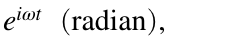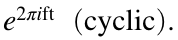Thus the relationship between the radian frequency ω and the cyclic frequency f is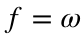/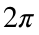.
I find it useful to work with both types of frequencies. Radian frequencies are convenient for theoretical expressions. However, cyclic frequencies are more intutive and therefore to be preferred when plotting or quoting values.
So now we redo the above plot in cyclic frequency, and covert from cycles per hour to cycles per day.
clf,
[f,spp,snn]=mspec(1/24,cv,[]); %Specify sampling interval dt in days
plot(f/2/pi,[spp snn]),axis tight,ylog,ylim(10.^[-3 4])
vlines(corfreq(lat)/2/pi*24,'2G--'),vlines(tidefreq/2/pi*24,':')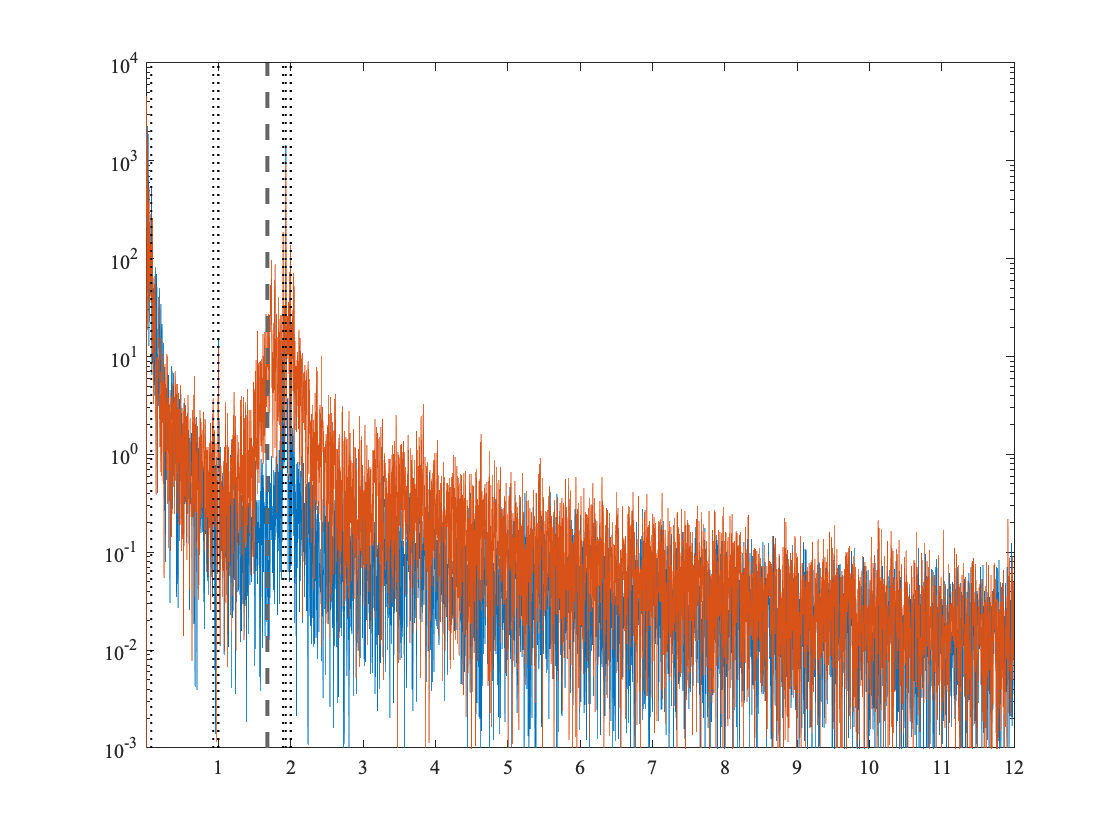Note that the semidiurnal tide is at 2 cycles per day, as expected. Changing to cycles per day has also changed the y-limits, on account of the normalization discussed earlier. Finally, it is often convenient to look at spectra with log/log axes:
clf,
[f,spp,snn]=mspec(1/24,cv,[]); %Specify sampling interval dt in days
plot(f/2/pi,[spp snn]),xlim([f(2) f(end)]/2/pi),xlog,ylog,ylim(10.^[-3 4])
vlines(corfreq(lat)/2/pi*24,'2G--'),vlines(tidefreq/2/pi*24,':')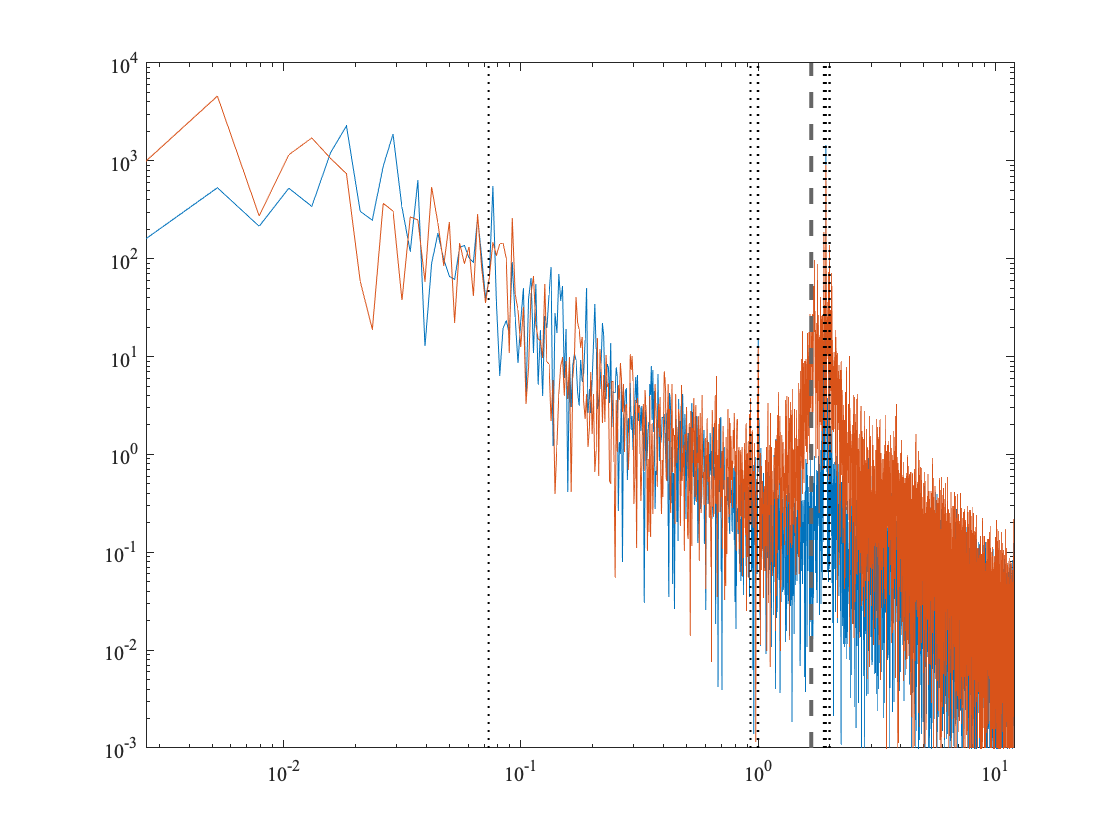Again we see the tidal frequencies appearing in three groups. Here, we can see that there are many more points at higher frequencies than at lower frequencies on account of the change to a logarithmic x-axis. The lowest nonzero frequency, the leftmost frequency appearing in this log-log plot, is an important quantity called the Rayleigh frequency that we have been discussing. The Rayleigh frequency in cyclic units is simply one over the length of the time series, and is equal to one cycle per 380 days or 0.0026 cycles per day for this time series.

# The Multitaper Method

The periodogram is now presented with meaningful units and on a meaningful frequency axis. However, we see that it has a very ‘fuzzy’ appearance on account of a high degree of variability. This high variance of the spectral estimate is a major, but not the only, problem with the periodogram. To remove this variance, we need to do some type of smoothing or averaging. The most convenient way to accomplish this is implicitly, through the use of a type of spectral estimate term the multitaper method.
First we will see how this works in practice, before exploring its theoetical basis. Here we will plot only the negative or clockwise side, which is in the output array snn from mspec. Also, we will try three different degrees of smoothing.
psi=sleptap(length(cv),4); %least smoothing
SLEPTAP calculating tapers of length 512.
SLEPTAP interpolating to length 9126.
[f,spp(:,2),snn(:,2)]=mspec(1/24,cv,psi);
psi=sleptap(length(cv),16); %intermediate smoothing
SLEPTAP calculating tapers of length 512.
SLEPTAP interpolating to length 9126.
[f,spp(:,3),snn(:,3)]=mspec(1/24,cv,psi);
psi=sleptap(length(cv),32); %most smoothing
SLEPTAP calculating tapers of length 512.
SLEPTAP interpolating to length 9126.
[f,spp(:,4),snn(:,4)]=mspec(1/24,cv,psi);
clf,
plot(f/2/pi,snn),xlim([f(2) f(end)]/2/pi),xlog,ylog,ylim(10.^[-3 4])
linestyle D T U k
vlines(corfreq(lat)/2/pi*24,'2G--')
vlines(tidefreq/2/pi*24,':')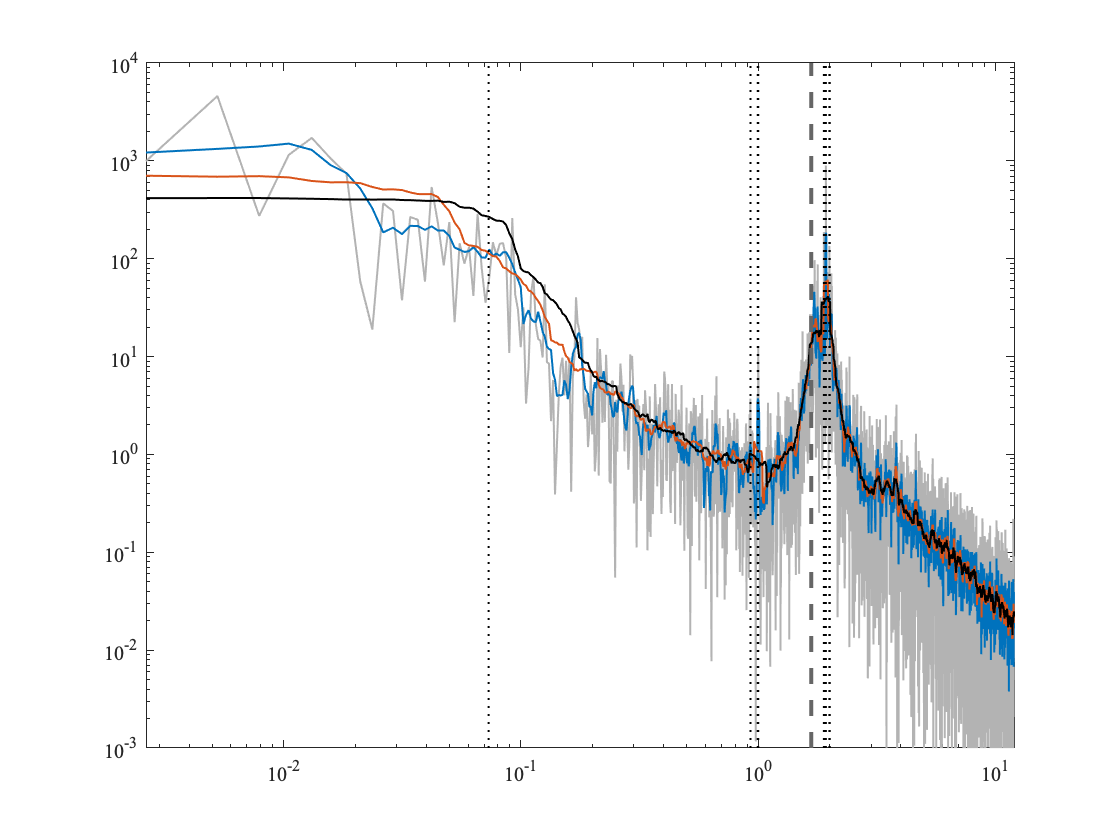The multitaper spectral estimate is implemented by mspec by first calling sleptap. This latter function computes a set of functions known as the Slepian tapers after their discoverer, David Slepian. More on these later. The important thing for the moment is the second argument to sleptap. When this quantity, known as the time-bandwidth product or P, is large, the spectral estimates are subject to a high degree of smoothing, and when it is small they are subject to a lesser degree of smoothing. Here, the gray line is the periodogram, and the blue, orange, and yellow curves are the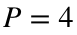,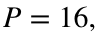and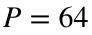multitaper estimates, respectively.
So in practical terms all you need to know to use the multitaper method is to increase the time-bandwidth product P until you feel you have obtained a suitable degree of smoothing. Deciding on an appropriate degree of smoothing is generally something that is done by ‘feel’, depending on what features you are interested in investigating, rather than something that has an objectively correct answer.
When the smoothing is too small, the spectral estimate is poor because of too much variance. When the smoothing is too large, the spectral estimate is poor not because it varies, but because it is systematically different from the true spectrum; this problem is called bias. These two problems trade off against each other. One of the challenges in signal analysis is trying to make a sensible choice in this bias-variance tradeoff.
Go ahead and pop this figure out, using the curved arrow at the right, and zoom into it. If you examine the semi-diurnal tidal peaks, the highest set of peaks around frequency 1.94, you will how the representation of this peak becomes more "blocky" as the smoothing increases. That's bias. It arises from the implicit frequency-domain smoothing that we are specifying in setting P. Again, we will understand why this bias occurs later; now we just need to know that it occurs.
To me the orange curve looks about right to resolve most of the spectral feautures, balancing reducing variance with over-smoothing. The black curve seems a bit oversmoothed to me. Therefore we will work now with this version, the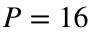multitaper estimate.
We will learn more about how the multitaper method works later. For now, I just want you to that it is very easy to use, simply by adjusting P. It is widely accepted that the multi-taper method is the best way of approaching spectral analysis. The idea was introduced in a landmark paper by David Thomson in 1982 that is one of the most influential papers in time series analysis of the 20th century.

# Rotary Spectra

We will now compare the positive and negative rotary spectra using themultitaper estimate.
psi=sleptap(length(cv),16);
SLEPTAP calculating tapers of length 512.
SLEPTAP interpolating to length 9126.
[f,spp,snn]=mspec(1/24,cv,psi);
clf,
plot(f/2/pi,[snn spp]),xlim([f(2) f(end)]/2/pi),xlog,ylog,ylim(10.^[-2 3])
linestyle T U
vlines(corfreq(lat)/2/pi*24,'2G--')
vlines(tidefreq/2/pi*24,':')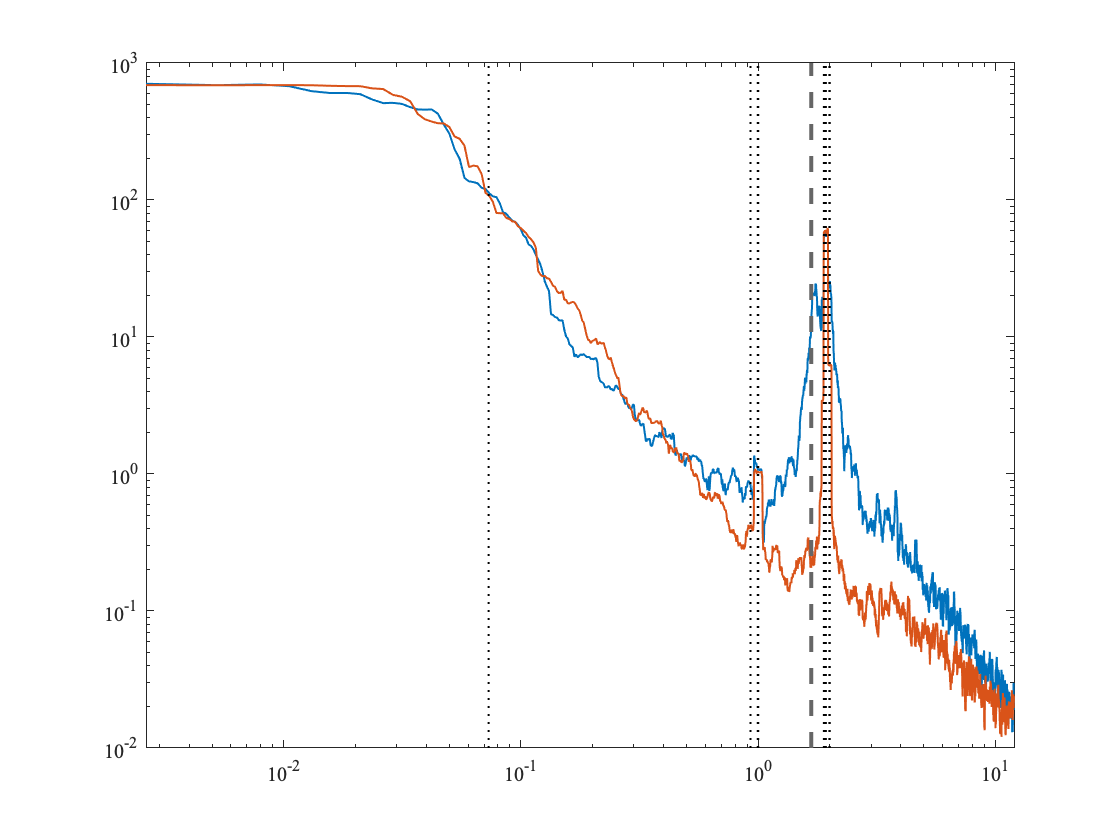Note the substantial differences between the two sides. On the negative side---the side that supports inertial oscillations in the Northern Hemisphere---we see a broad wave band surrounding the semidiurnal tide. These are internal waves clustered around the inertial frequency. On the positive side, there is a very narrow semidiurnal peak. Such a narrow peak is called narrowband as opposed to the broadband peak on the positive side.
If you pop the window out and zoom in, you can see that at this narrowband peak, the blue and orange curves are almost identical. This is hard to see without zooming in because the orange curve is obscuring the blue one. The fact that the blue and orange curves are of similiar magnitude means that this signal is roughly linearly polarized---the currents trace out a line as they oscillate, not a circle as would be the case for the broadband peak, which is almost nonexistent on the positive side.
On the negative frequency side, the narrowband peak is also obscured by the broadband peak. But when we zoom in we can clearly see that the narrowband peaks stiicks up above the broadband peak. If you change to a linear y-axis with ylin, you will see that the narrowband peak is more than twice the magnitude of the broadband peak.
The reason that the orange peak is so narrow is that free waves are not supported on this side. (We know this from our physical understanding of the internal wave dispersion relation.) This leads us to hypothesize that the narrow peak on the positive side is the barotropic tide, not the baroclinic tide associated with excited internal waves. This could be investigated more by looking at the vertical shear in this frequency band on the two sides of the spectrum. On the negative frequency tide, because the inertial and semidiurnal frequencies are so close together at this latitude, we can't really distinguish the background internal wave spectrum from free internal waves excited by the barotropic tide. At other latitudes, you can.
Moving towards lower or subinertial frequencies, the positive and negative spectra quickly converge, due to the fact the free internal waves are no longer supported below the Coriolis frequency. At higher frequencies, however, the negative spectrum becomes closer to, but remains stronger than, the positive spectrum up to the highest resolvable frequency. This behavior is a direct consequence of the known polarization behavior for internal waves.
Given the inertial frequency f and the buoyancy frequency N, the horizontal currents due to internal waves form an ellipse, the eccentricity of which is known as a function of frequency. This ellipse becomes a circle at f where the internal waves become pure inertial oscillations, and a straight line at N, where the waves become pure internal gravity waves. Thus, what we are seeing here is the internal wave spectrum becoming more linearly polarized and less circularly polarized---more like gravity waves and less like inertial oscillations---as we head towards the bouyancy frequency.

# Cartesian Spectra

Next we will compare the rotary spectra with the Cartesian spectra. We will learn more about this in class, however, the basic idea is that we have a choice as to how to split up the spectrum of our complex-valued velocity signal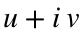. We can choose to look at the rotary spectrum, separating positive and negative frequencies, or alternatively, we can choose to look at the spectra of u and v considered separately. In this latter case, the positive and negative frequency sides of u are grouped together, as are the positive and negative frequency sides of v. We refer to the resulting pair of one-sides spectra as the Cartesian spectra, to distinguish these from the rotary spectra.
The Cartesian spectra and rotary spectra are shown in the following block. Here blue and orange are the eastward and northward Cartesian spectra, while gray and black are the positive and negative rotary spectra.
use bravo94.rcm
cv=cv(:,6);
cv=cv-mean(cv);
[f,sxx,syy]=mspec(1/24,real(cv),imag(cv),psi);
clf,
plot(f/2/pi,[sxx syy spp snn]),xlim([f(2) f(end)]/2/pi),xlog,ylog,ylim(10.^[-2 3])
linestyle T U D k
vlines(corfreq(lat)/2/pi*24,'2G--')
vlines(tidefreq/2/pi*24,':')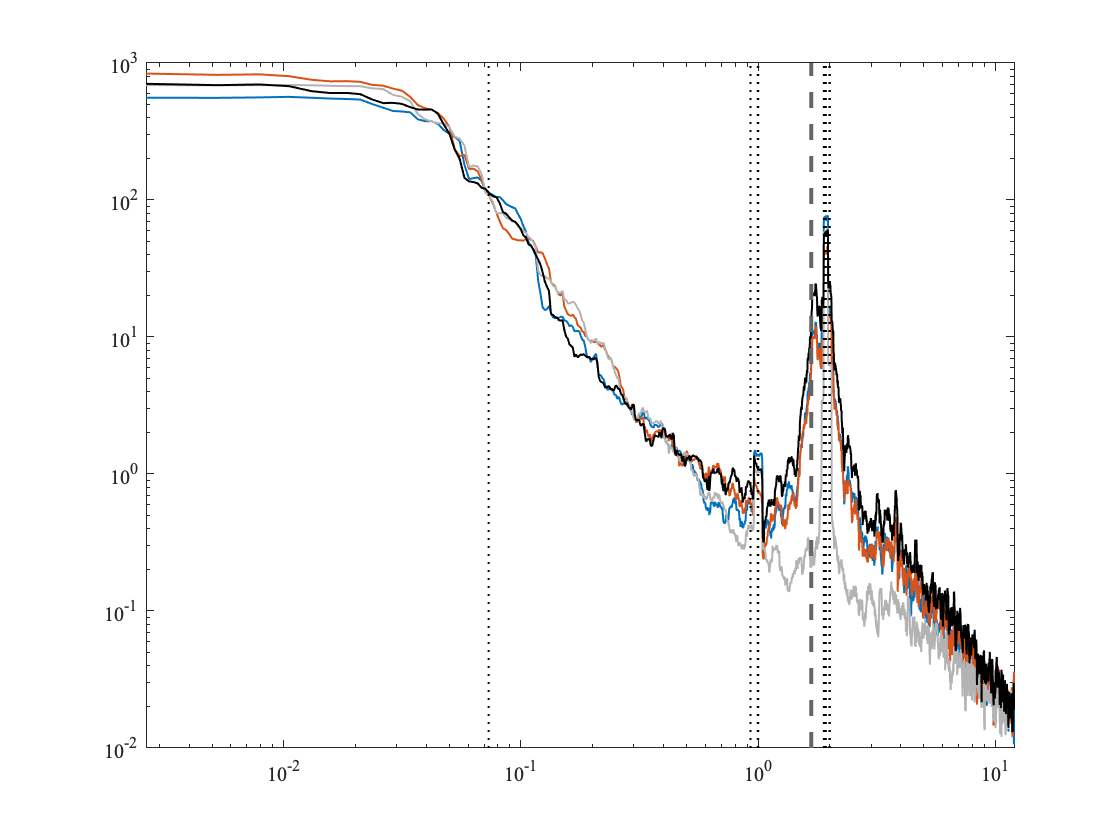A rule of thumb is that a phenomena is most compactly described by the spectral pair exhibiting the highest degree of anisotropy. In the inertial band, we see a much higher degree of anisotropy for the rotary pairing than for the Cartesian pairing. This occurs because the near-inertial band consists of nearly circular ellipses. Here, we see that the near-inertial variability projects strongly onto both Cartesian components.
There are other examples of phenomenon that exhibit asymmetry in the Cartesian domain, most notably, boundary current variaibility. Whether the rotary or Cartesian perspective on the spectra is most suitable is not a property of the time series. Rather is it a property of the phenomenon. Even within one time series, we may need to use different perspectives to best analyze phenomena at different frequencies. In general, you should always look at the rotary spectrum, a also the Cartesian spectra rotated either to align with the bathymetry, or the mean current, or the direction of highest variance.

# Spectra of Discontinuous Signals

In this course we have been looking a lot at velocity spectra, and not much at spectra of temperature and salinity. There is a reason for this. Often in oceanography, temperature and salinity at fixed depths have a discontinuous appearance on account of the mixed layer passing that depth. You really do not want to take spectra of such data, as this example illustrates.
t=[-100:.1:100]';
x=cos(2*pi*t/10);
x(:,2)=sign(x);
figure,plot(t,x),ylim([-1.1 1.1])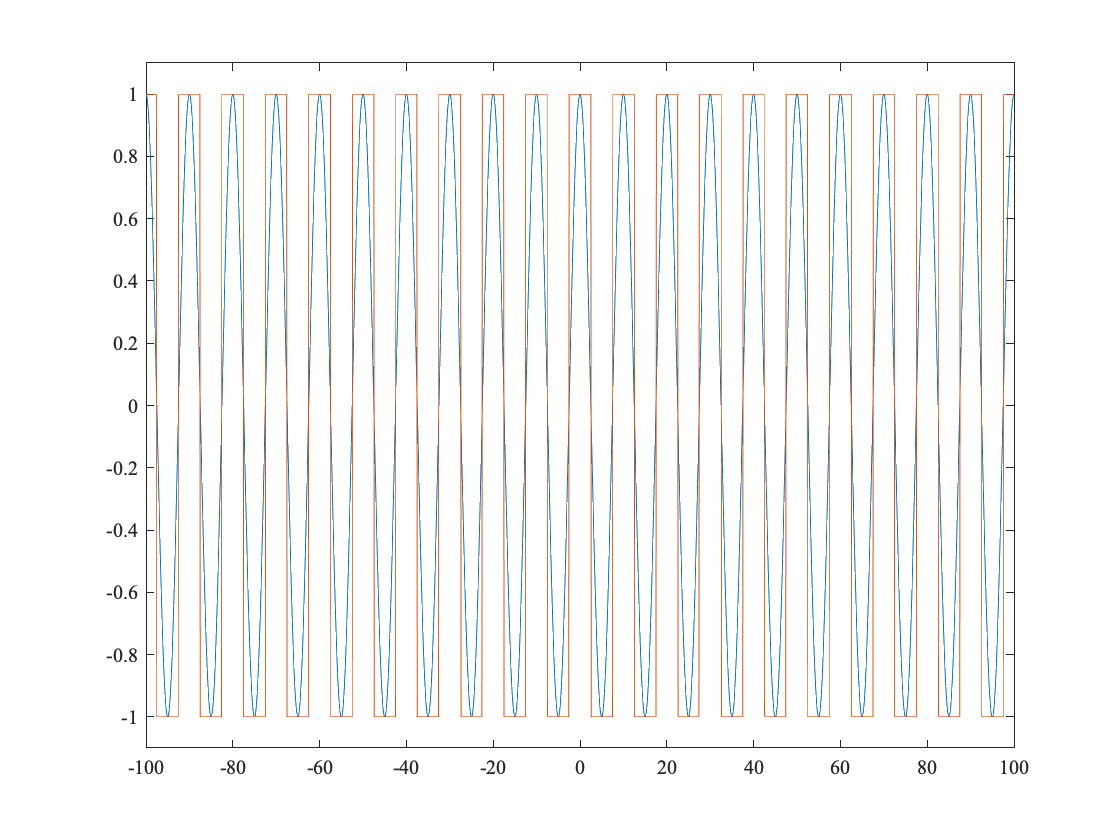Here we have created two signals, a cosine and a square wave having the same period as the cosine. Let's look at their squared Fourier transforms or periodograms.
clf, plot(squared(fft(x))),axis tight,ylog,ylim(10.^[1 7])
linestyle 4T U